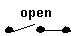#### Intro to Binary Numbers

Computers handle and store data using a binary number system.  This system of numbers is a base-2 number system.
In htis type of system there are only 2 digits; hence the name "binary" derived from the Latin words meaning "two numbers".
In our decimal number system there are 10 digits.
The two digits used in the binary number system are the integer (zero) and the interger 1 (one).
All other numbers on this system are based on these two numbers only: a series of zeros and ones ca be used to represent any decimal equivalent number.

For example, the equivaent number for year 2020 in binary digits is 11111100100.  We will discuss later how to convert from one system to the other.
The reason why computers use this system instead of the decimal number system is that it is easier and less expensive to build computers components (hardware) that use a logic based on only two digits instead of ten.
In Computer Science we use the word BIT which is a short-form of the phrase Binary Digit, to indicate a basic unit of information.
The numbers one and zero correspond to the two possibe logic states of a switch.
• When the switch is open or OFF, no current flows through it and therefore its state is OFF or equivalent to ZERO.... ZERO STATE --- Binary Digit 0
• When the switch is closed or ON, current flows through it and terefore its state is ON or equivalent to ONE... ONE STATE  --- Binary Digit 1

Below are the fiist 10 numbers in the decimal system compared to their equivalent numbers in the binary system.

Decimal -- Binary
0           =     0
1           =     1
2           =    10
3           =    11
4           =   100
5           =   101
6           =   110
7           =   111
8           =  1000
9           =  1001
10          =  1010

Activity: Try to figure out how to covert from one system to the other?